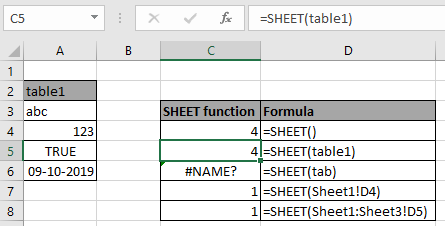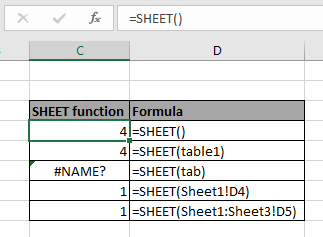# How to use the SHEET Function in ExcelWhat is the SHEET function?

SHEET function in excel is a built-in reference function. This function returns a number, reference to the input sheet indicated. SHEET function can be used for the following purposes in Excel.

1. The function returns the total number of sheets in the current worksheet.
2. The function returns the sheet number of the reference sheet cell.
3. The function returns the sheet number for the reference table in the worksheet.

What is the SHEET function?

SHEET function in excel returns SHEET number for the reference value.

Syntax of SHEET function:

= SHEET ( value )

value : value be given using cell reference

Example:

All of these might be confusing to understand. So, let's test this formula via running it on the example shown below. Here we have some random data values to test the SHEET function in Excel.

Here we have some different uses of SHEET function and we need to get the Sheet number in number format .using the below formula

Use the formula:

=SHEET()

Or

=SHEET(table1)

Where table1 is name given to the table using the named range in Excel.As you can see the formula returns error if the table name is not defined.

Here are some observational notes shown below.

Notes:

1. The formula returns the sheet number of the input reference cell.
2. If the sheet array is given as cell reference, the formula returns the first sheet number.
3. Use the SHEETS function to count the number of sheet if the range of sheet is given as input.

Hope this article about How to use the SHEET function in Excel is explanatory. Find more articles on the SHEETS function here. If you liked our blogs, share it with your fristarts on Facebook. And also you can follow us on Twitter and Facebook. We would love to hear from you, do let us know how we can improve, complement or innovate our work and make it better for you. Write to us at T@exceltip.com.

Related Articles

How to use the CELL function in Excel : returns Information about a cell in a worksheet using the CELL function.

How to use the TYPE Function in Excel : returns numeric code representing the TYPE of data using TYPE function in Excel.

How to use the SHEETS function in Excel : returns a number, reference to input sheet indicated using the SHEETS function.

How to Use ISNUMBER Function in Excel : ISNUMBER function returns TRUE if number or FALSE if not in Excel.

Popular Articles:

50 Excel Shortcuts to Increase Your Productivity | Get faster at your task. These 50 shortcuts will make you work even faster on Excel.

The VLOOKUP Function in Excel | This is one of the most used and popular functions of excel that is used to lookup value from different ranges and sheets.

COUNTIF in Excel 2016 | Count values with conditions using this amazing function. You don't need to filter your data to count specific values. Countif function is essential to prepare your dashboard.

How to Use SUMIF Function in Excel | This is another dashboard essential function. This helps you sum up values on specific conditions.

Terms and Conditions of use

The applications/code on this site are distributed as is and without warranties or liability. In no event shall the owner of the copyrights, or the authors of the applications/code be liable for any loss of profit, any problems or any damage resulting from the use or evaluation of the applications/code.